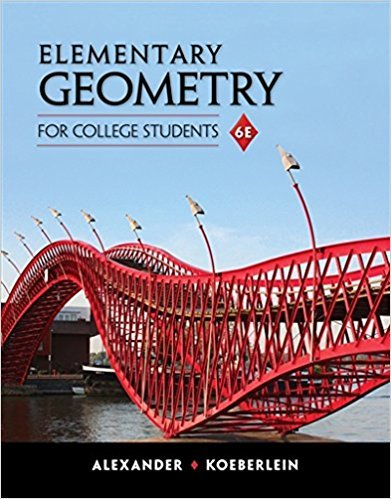×
Log in to StudySoup
Get Full Access to Math - Textbook Survival Guide
Join StudySoup for FREE
Get Full Access to Math - Textbook Survival Guide

Already have an account? Login here
×
Reset your password

Textbooks / Math / Elementary Geometry for College Students 6

# Elementary Geometry for College Students 6th Edition - Solutions by Chapter## Full solutions for Elementary Geometry for College Students | 6th Edition

ISBN: 9781285195698Elementary Geometry for College Students | 6th Edition - Solutions by Chapter

Solutions by Chapter
4 5 0 353 Reviews
##### ISBN: 9781285195698

Elementary Geometry for College Students was written by and is associated to the ISBN: 9781285195698. Since problems from 11 chapters in Elementary Geometry for College Students have been answered, more than 5419 students have viewed full step-by-step answer. This expansive textbook survival guide covers the following chapters: 11. The full step-by-step solution to problem in Elementary Geometry for College Students were answered by , our top Math solution expert on 01/29/18, 03:43PM. This textbook survival guide was created for the textbook: Elementary Geometry for College Students, edition: 6.

Key Math Terms and definitions covered in this textbook
• Change of basis matrix M.

The old basis vectors v j are combinations L mij Wi of the new basis vectors. The coordinates of CI VI + ... + cnvn = dl wI + ... + dn Wn are related by d = M c. (For n = 2 set VI = mll WI +m21 W2, V2 = m12WI +m22w2.)

• Characteristic equation det(A - AI) = O.

The n roots are the eigenvalues of A.

• Circulant matrix C.

Constant diagonals wrap around as in cyclic shift S. Every circulant is Col + CIS + ... + Cn_lSn - l . Cx = convolution c * x. Eigenvectors in F.

• Cramer's Rule for Ax = b.

B j has b replacing column j of A; x j = det B j I det A

• Cross product u xv in R3:

Vector perpendicular to u and v, length Ilullllvlll sin el = area of parallelogram, u x v = "determinant" of [i j k; UI U2 U3; VI V2 V3].

• Dot product = Inner product x T y = XI Y 1 + ... + Xn Yn.

Complex dot product is x T Y . Perpendicular vectors have x T y = O. (AB)ij = (row i of A)T(column j of B).

• Eigenvalue A and eigenvector x.

Ax = AX with x#-O so det(A - AI) = o.

• Free columns of A.

Columns without pivots; these are combinations of earlier columns.

• Full column rank r = n.

Independent columns, N(A) = {O}, no free variables.

• Gram-Schmidt orthogonalization A = QR.

Independent columns in A, orthonormal columns in Q. Each column q j of Q is a combination of the first j columns of A (and conversely, so R is upper triangular). Convention: diag(R) > o.

• Hermitian matrix A H = AT = A.

Complex analog a j i = aU of a symmetric matrix.

• Krylov subspace Kj(A, b).

The subspace spanned by b, Ab, ... , Aj-Ib. Numerical methods approximate A -I b by x j with residual b - Ax j in this subspace. A good basis for K j requires only multiplication by A at each step.

• Linearly dependent VI, ... , Vn.

A combination other than all Ci = 0 gives L Ci Vi = O.

• Multiplication Ax

= Xl (column 1) + ... + xn(column n) = combination of columns.

• Reflection matrix (Householder) Q = I -2uuT.

Unit vector u is reflected to Qu = -u. All x intheplanemirroruTx = o have Qx = x. Notice QT = Q-1 = Q.

• Saddle point of I(x}, ... ,xn ).

A point where the first derivatives of I are zero and the second derivative matrix (a2 II aXi ax j = Hessian matrix) is indefinite.

• Semidefinite matrix A.

(Positive) semidefinite: all x T Ax > 0, all A > 0; A = any RT R.

• Singular matrix A.

A square matrix that has no inverse: det(A) = o.

• Symmetric matrix A.

The transpose is AT = A, and aU = a ji. A-I is also symmetric.

• Triangle inequality II u + v II < II u II + II v II.

For matrix norms II A + B II < II A II + II B II·